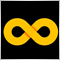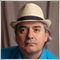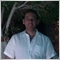# Baffled by Floating Point numbers?37973

Hi, im having trouble understanding why im getting the following return.

In the following code :

`long number=(long)(0.9553700000000001*MathPow(10,16));`

number = 9553700000000000

But when i move the 1st digit down to 8 as follows :

`long number=(long)(0.8553700000000001*MathPow(10,16));`

number =  8553700000000001

The last digit is 1

It seems whenever number is at 0.9 or above it returns 0 at end but, at or below 0.8 it returns 1 at end?

Very odd theres something i dont understand here.6954

Stephen Reynolds: Hi, im having trouble understanding why im getting the following return. Very odd theres something i dont understand here. Can someone please explain

Do a search on the forum. There are many, many threads about this as most coders (especially newbie coders) don't understand that floating-point is represented in binary form which cannot equate to exact decimal values in all cases.

Do do the search and read-up on the information presented.25844

Floating-point has an infinite number of decimals, it's you, not understanding floating-point and that some numbers can't be represented exactly. (like 1/10.)
Double-precision floating-point format - Wikipedia

If you want to see the correct number of digits, convert it to a string with the correct/wanted accuracy.
question about decima of marketinfo() - MQL4 programming forum 2016.05.1837973

Fernando Carreiro:

Do a search on the forum. There are many, many threads about this as most coders (especially newbie coders) don't understand that floating-point is represented in binary form which cannot equate to exact decimal values in all cases.

Do do the search and read-up on the information presented.

Thanks I will look into this more37973

William Roeder:

Floating-point has an infinite number of decimals, it's you, not understanding floating-point and that some numbers can't be represented exactly. (like 1/10.)
Double-precision floating-point format - Wikipedia

If you want to see the correct number of digits, convert it to a string with the correct/wanted accuracy.
question about decima of marketinfo() - MQL4 programming forum 2016.05.18

thanks okay i will look into this4751

This is how floating-point doubles work. All numbers >= 2^53 (9007199254740992) are even integers. Odd numbers greater than 2^53 are rounded to the nearest even.

You can use this link to check it: https://baseconvert.com/ieee-754-floating-point

Base Convert: IEEE 754 Floating Point
• baseconvert.com
Online IEEE 754 floating point converter and analysis. Convert between decimal, binary and hexadecimal